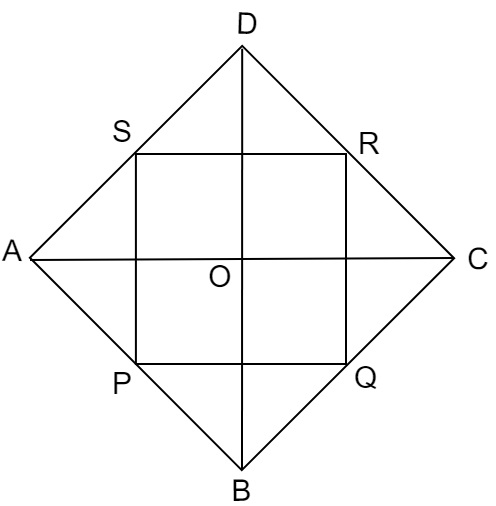# $\mathrm{ABCD}$ is a rhombus and $\mathrm{P}, \mathrm{Q}, \mathrm{R}$ and $\mathrm{S}$ are the mid-points of the sides $\mathrm{AB}, \mathrm{BC}, \mathrm{CD}$ and DA respectively. Show that the quadrilateral $\mathrm{PQRS}$ is a rectangle.

Given:

$\mathrm{ABCD}$ is a rhombus and $\mathrm{P}, \mathrm{Q}, \mathrm{R}$ and $\mathrm{S}$ are the mid-points of the sides $\mathrm{AB}, \mathrm{BC}, \mathrm{CD}$ and DA respectively.

To do:
We have to show that the quadrilateral $\mathrm{PQRS}$ is a rectangle.
Solution:

Join $PQ,QR,RS,PS,AC$ and $BD$.In $\triangle DRS$ and $\triangle BPQ$,

$DS = BQ$                 ($\frac{AD}{2}=\frac{BC}{2}$)

$\angle SDR = \angle QBP$         (Opposite angles of a rhombus are equal to each other)

$DR = BP$                   ($\frac{CD}{2}=\frac{AB}{2}$)

Therefore, by SAS congruency, we get,

$\triangle DRS \cong \triangle BPQ$

This implies,

$RS = PQ$           (CPCT)............(i)

$In \triangle CQR$ and $\triangle ASP$,

$RC = PA$           ($\frac{CD}{2}=\frac{AB}{2}$)

$\angle RCQ = \angle PAS$             (Opposite angles of the rhombus)

$CQ = AS$           ($\frac{BC}{2}=\frac{AD}{2}$)

Therefore, by SAS congruency, we get,

$\triangle QCR \cong \triangle SAP$

This implies,

$RQ = SP$         (CPCT)...............(ii)

In $\triangle CBD$,

$R$ and $Q$ are the mid points of $CD$ and $BC$ respectively.

This implies,

$QR \| BD$

In $\triangle ABD$,

$P$ and $S$ are the mid points of $AD$ and $AB$ respectively.

This implies,

$PS \| BD$

Therefore,

$QR \| PS$............(iii)

From (i), (ii) and (iii), we get,

$PQRS$ is a parallelogram.

$AB$ is a straight line.

$\angle APS + \angle SPQ + \angle QPB = 180^o$

$BC$ is a straight line.

$\angle PQB + \angle PQR + \angle CQR = 180^o$

$\angle APS + \angle SPQ + \angle QPB = \angle PQB + \angle PQR + \angle CQR$

$\angle APS + \angle SPQ + \angle QPB = \angle QPB + \angle PQR + \angle APS$

$\angle SPQ = \angle PQR$

$\angle SPQ + \angle PQR = 180^o$        (Adjacent angles of a parallelogram are supplementary)

$2\angle PQR = 180^o$

$PQR = 90^o$

In $PQRS$,

$RS = PQ$

$RQ = SP$

$\angle Q = 90^o$

Therefore,

$PQRS$ is a rectangle.

Updated on: 10-Oct-2022

33 Views